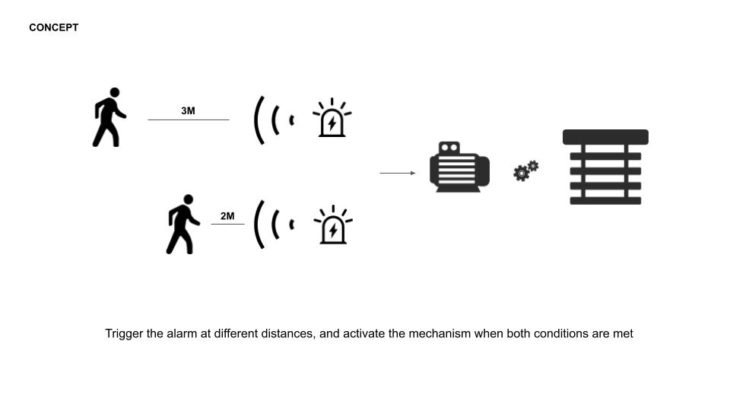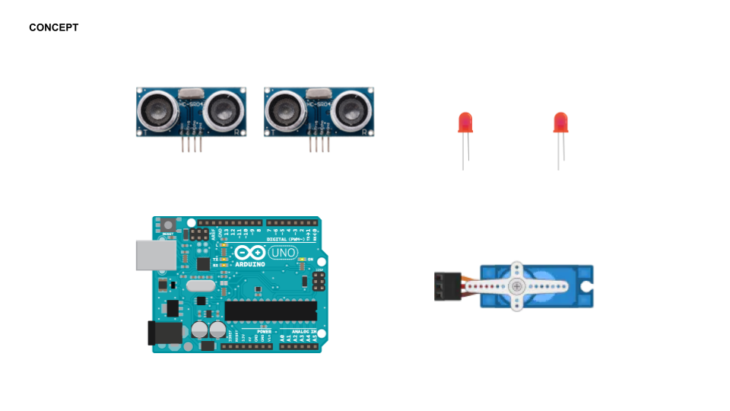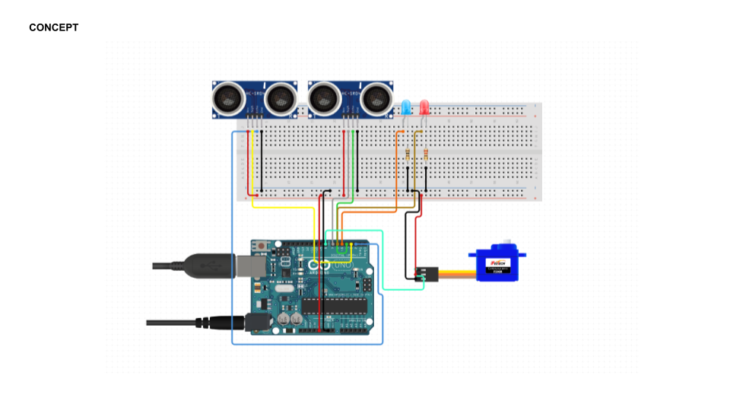Introduction to Programming and Physical Computing

CHANGKAI QI

It’s a double safety device that activates something through a double confirmation

It can be used for the entrance of important places. When two sonar meet the distance condition at the same time, the door will open

Or a second warning，When people are 30M away from danger, the blue light will be on. When people are close in 20M, the red light will be on. When people are very close in 10M, the motor will work and raise the fenceint ledPin1 = 3;
int ledPin2 = 4;

int trigPin1 = 6;
int echoPin1 = 7;

int trigPin2 = 8;
int echoPin2 = 9;
#include <Servo.h>
Servo myservo;
#define servoPin 11
int angle = 0;

void setup() {
Serial.begin (9600);
pinMode(trigPin1, OUTPUT);
pinMode(echoPin1, INPUT);

pinMode(trigPin2, OUTPUT);
pinMode(echoPin2, INPUT);

pinMode(ledPin1, OUTPUT);
pinMode(ledPin2, OUTPUT);

Serial.begin(9600);
myservo.attach(servoPin);

}

void firstsensor(){ //
int duration1, distance1;
digitalWrite (trigPin1, HIGH);
delayMicroseconds (10);
digitalWrite (trigPin1, LOW);
duration1 = pulseIn (echoPin1, HIGH);
distance1 = (duration1/2) / 29.1;

Serial.print(“1st Sensor: “);
Serial.print(distance1);
Serial.print(“cm “);

if (distance1 < 30) {
digitalWrite (ledPin1, HIGH);
} else {
digitalWrite (ledPin1, LOW);
}
}
void secondsensor(){
int duration2, distance2;
digitalWrite (trigPin2, HIGH);
delayMicroseconds (10);
digitalWrite (trigPin2, LOW);
duration2 = pulseIn (echoPin2, HIGH);
distance2 = (duration2/2) / 29.1;

Serial.print(“2nd Sensor: “);
Serial.print(distance2);
Serial.print(“cm “);

if (distance2 < 20) {
digitalWrite (ledPin2, HIGH);
}
else {
digitalWrite (ledPin2, LOW);
}

digitalWrite (trigPin2, HIGH);
delayMicroseconds (10);
digitalWrite (trigPin2, LOW);
duration2 = pulseIn (echoPin2, HIGH);
distance2 = (duration2/2) / 29.1;

Serial.print(“2nd Sensor: “);
Serial.print(distance2);
Serial.print(“cm “);

if (distance2 < 10) {

myservo.write(90);
delay(1000);
myservo.write(180);
delay(1000);
myservo.write(0);
delay(1000);

for (angle = 0; angle <= 180; angle += 1) {
myservo.write(angle);
delay(15);
}

for (angle = 180; angle >= 0; angle -= 1) {
myservo.write(angle);
delay(30);
}
delay(1000);

}

}
void loop() {
Serial.println(“\n”);
firstsensor();
secondsensor();

delay(100);
}

(Double Confirmation Device) is a project of IaaC, Institute for Advanced Architecture of Catalonia developed at ( INTRODUCTION TO PROGRAMMING AND PHYSICAL COMPUTING) in (2021/2022) by: Students: (Changkai Qi) Faculty: (BERNAT MORATO, DANIIL KOSHELYUK)